# Deep Learning a Monty Hall Strategy (or, a gentle introduction to Deep-Q learning and OpenAI Gym with PyTorch)

## Deep Learning a Monty Hall Strategy (or, a gentle introduction to Deep-Q learning and OpenAI Gym with PyTorch)

(Read the original on Towards Data Science)

You never know how a combination of events is going to affect you.

For me, bingeing an old episode of Brooklyn-99, combined with Tensorflow’s recent announcement that they’ve officially incorporated the Edward probabalistic programming library, set me to thinking about Bayesian probability for the first time in a while.

In the episode, Captain Holt and his husband are arguing about the Monty Hall problem.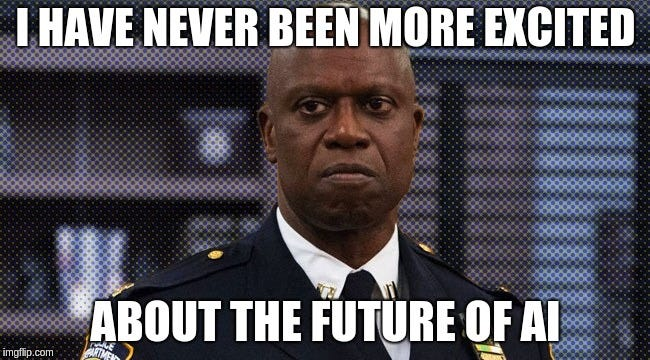I’m not familiar with the old gameshow, but the formulation of the problem is something like this:

There are three doors, behind one is a car, and behind the other two are goats. Pick one. Once you have picked, Monty, the gameshow host, will open one of the two doors you didn’t pick — one that definitely does not have the car behind it. Should you stick with your original guess, or switch to the other of the two doors?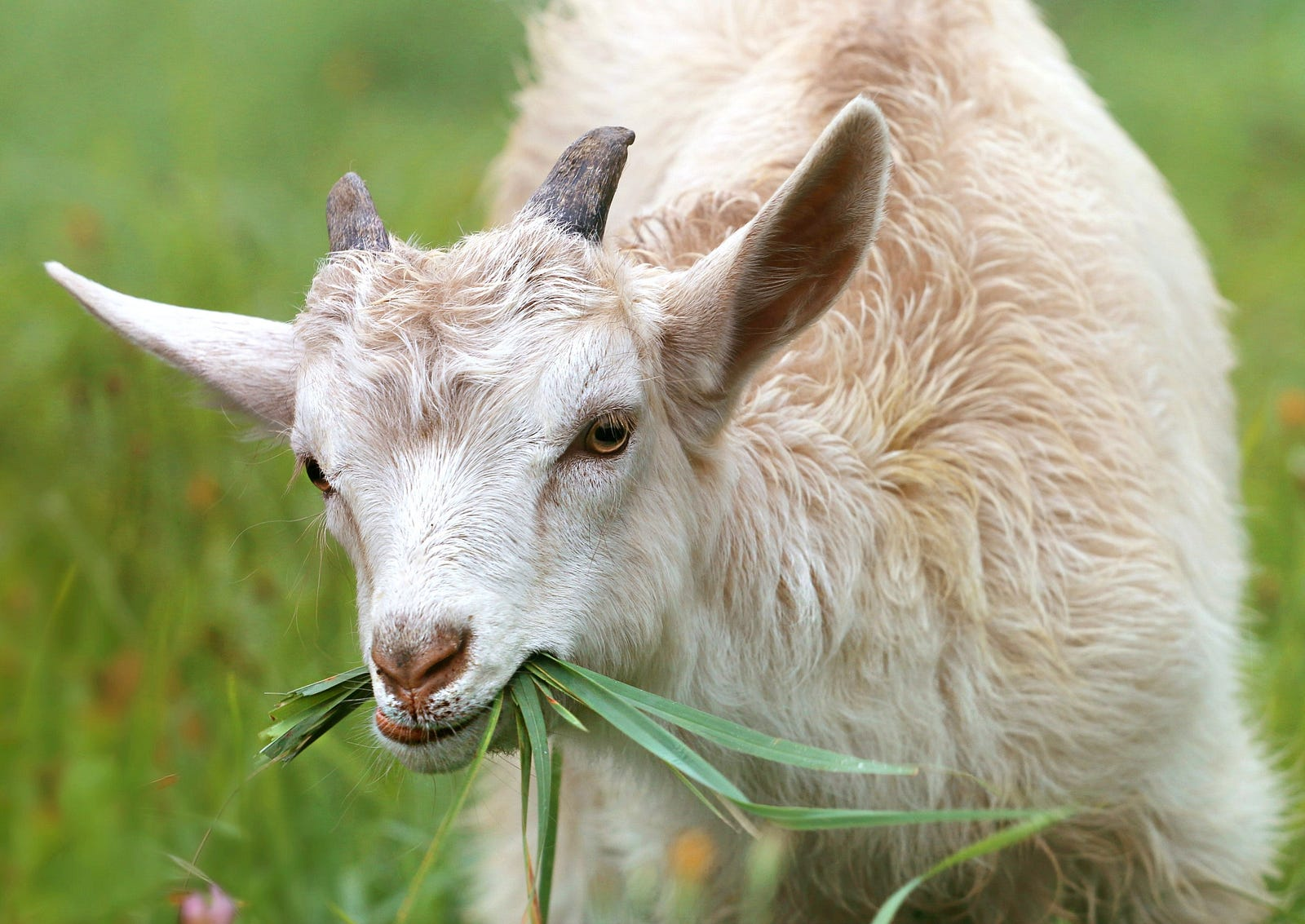Obviously, each door has a 13 chance of winning, and that doesn’t change after Monty opens a door. The Bayesian prior is [13, 13, 13]. Once a losing door is opened however, there is new information and the probabilities should be re-examined. In fact, the probability of the car being behind the unchosen door now ‘concentrates’ to 23, absorbing the probability of the dud door that Monty opened. That is, the posterior is [13, 0, 23]. Thus, you are unlikely to always win, but you do double your chances by switching to the unopened door you didn’t pick originally.

And that is the dominant strategy for this game.

If you want to skip straight to the relatively simple Jupyter Notebook that accompanies this article, it’s here.

### Where This Is Going

Most of my past work with AI has been in regression with a bit of generative stuff, and I’ve stayed away from the headline making techniques: AI teaches itself to play Doom, AI now better than humans at Go, AI self-driving cars self-organize into Autobots and Decepticons, etc. Because of this, I haven’t had the chance to play with Open AI Gym either. As it turns out, both are really easy to use, and I think deserving of a place in the everyman’s deep learning toolbox.

As usual for my writing I’ll leave out most of the math. There’s some involved in Deep-Q learning, and though it’s not complicated, I can tell you from my thesis work that random practical implementations come first, and the math is a nice post-hoc proof of completeness that enables you to write a serious paper. Intuition is the important bit.

I’m not actually going to get to trainable distributions and Tensorflow’s Edward in this article, but instead show how to build a simple, scaleable agent for solving a game theory-type problem that can interact with today’s cutting edge AI environment.

## Open AI Gym

Let’s get this one out of the way first. There isn’t any magic to the Gym, just a nice, standardized set of environments in which to test autonomous AI agents, simulating the effects of their actions on the environment. It’s installed just via pip install gym, and it’s easily used inside Jupyter Notebook.

One of the very simple environments that comes with the Gym is called CartPole, and it looks like this: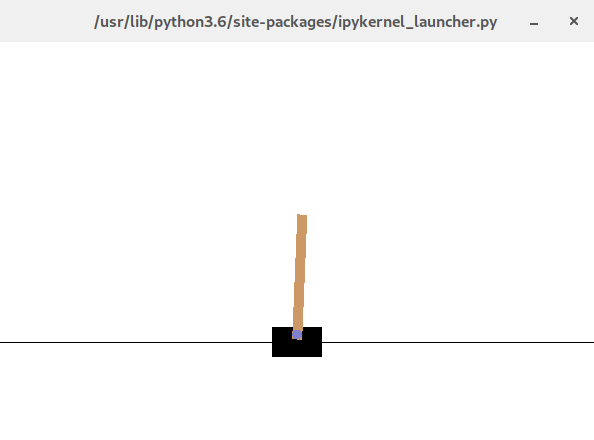The pole’s attached via a pivot to a cart on a track, and the objective is for the cart to keep the pole upright — imagine trying to balance a pen on your palm.

The environment simulates forces and gravity, and the action space of the environment is that the cart can be pushed left or right (the track is frictionless, so any pushing will increase the cart’s velocity, then it will continue at that velocity). The game ends when the pole falls over past an angle of no return.

The action space defines the dimensionality of the output of the AI (which I’ll assume is a neural net); there are two possible actions at each moment. Accordingly, you might have a single output neuron where an output > 0 is interpreted as push right and ≤ 0 as push left. More likely, you might have two neurons corresponding to left and right, and take the argmax of them.

Conversely, the observation space of the environment forms the input to the AI. Any AI trying to solve CartPole gets four inputs: floats representing velocities and angles.

Often when you hear Open AI Gym referred to, people are talking about Atari games: the action space would be the buttons on a gamepad, and the observation space would be the pixels on screen in a game of Asteroids. Here, I’m solving a simple game theory problem, but in a standardized way that’s compatible with (i.e., subclasses) the Gym.

Using the Gym in a typical PyTorch training loop looks something like this:


import gym
env = gym.make("CartPole-v0")
model = MyAI()
env.reset()
for frame in range(0, 6000): # frames of the game to play out
action = model.act(state, epsilon)
next_state, reward, done, _ = env.step(action)
replay_buffer.append(state, action, reward, next_state, done)
state = next_state
env.render()
if done:
env.reset()
if len(replay_buffer) > batch_size: # won or died
loss = calc_loss_over_episode(replay_buffer)
loss.backward()
optimizer.step()


There are a few things to unpack here, before even getting to Deep-Q learning:

• MyAI is a placeholder for a neural network model, built in a standard PyTorch way as will follow, but with an additional act method.
• Resetting the Gym environment, predictably, sets it back to an initial state.
• Epsilon is a number that decays with time, as in simulated annealing (more below).
• Each step in the environment returns a new state, a reward (which could be zero until the game is won), a flag to say if we are done (won or lost, in which case reset the environment), and optionally some debug info.
• env.render() just does the graphics. Gym provides some helpful primitives for graphics, if you want to make your own.
• Losses are averaged over an episode or batch, which may contain one or more complete games. All the action that took place in that time is stored in a replay buffer, enabling it to be played back — so we may calculate loss as we hopefully moved closer to the goal — at the end of the episode.
• calc_loss_over_episode will be covered in the Deep-Q bit below!

## The Environment

Creating a gym for Open AI is easy. You just have to subclass the gym and implement a few methods. Here’s a template:


from gym import spaces
from gym.envs.classic_control import rendering
out = 3
in = 3
class MyEnv(gym.Env):
def __init__(self):
self.action_space = spaces.Discrete(out)
self.observation_space = spaces.Discrete(in)
self.initial_state = whatever_you_like
self.state = self.initial_state
self.viewer = rendering.Viewer(640, 480)
def step(self, action):
# do something to calculate next state, reward, and done
return self.state, reward, self.done, {}
def reset(self):
self.state = initial_state
self.done = False
return self.state
def render(self, mode='human'):
# This gets you a window and primitives e.g.
# cart = rendering.FilledPolygon([(l,b), (l,t), (r,t), (r,b)])
return self.viewer.render()


The action space needs to be discrete because each action will be 0 or 1 (take, don’t take). The observation space is potentially wider. It depends on how you formulate the problem, and here’s how I formulated Monty Hall: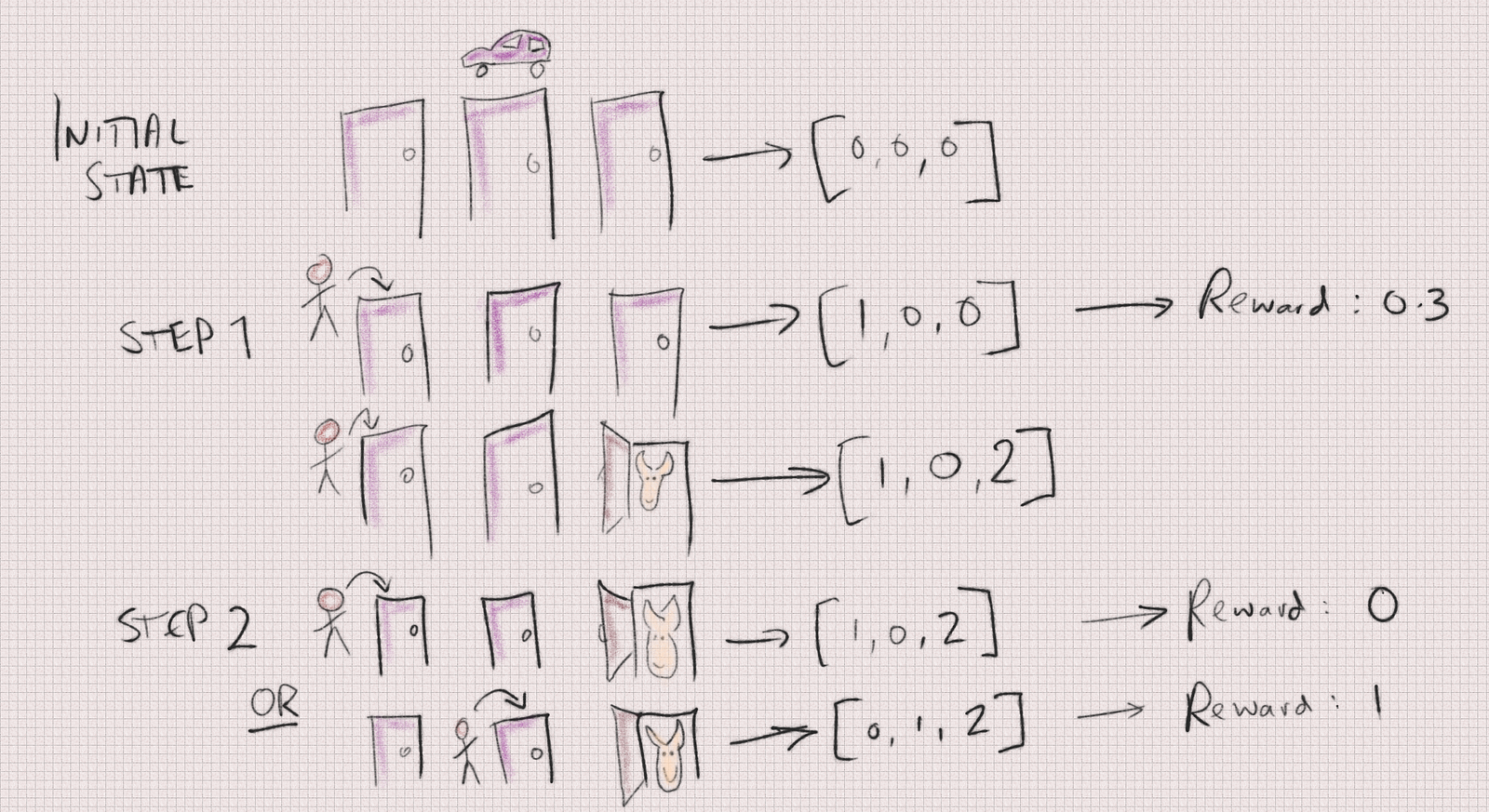Believe it or not, formulating the problem in a suitable way for AI was the most difficult part of putting together this article.

First, there’s a hidden state, initialized when the game resets (after every two moves). The hidden state just says which door the car is behind; the other two doors contain goats. The observation state, visible to the AI, starts out as [0,0,0]. Next, the AI picks a door, an action for which it gets a constant reward of 0.3. (I chose this number arbitrarily; rewards can be any positive or negative real numbers, with the AI tasked to maximize it).

The state then changes when Monty opens a door; this is indicated to the AI by a change in the observed state, marking the newly opened (and goat-containing) door with a 2. Next the AI takes a second action: it can choose the opened door (fail!), stick with its original guess, or twist. That ends the game, and if the AI chose the correct door ultimately it gets +1 reward. If not, it gets 0 reward. As we know it’s better, most of the time, to twist: changing its original guess to the other unopened door.

So, what the AI sees: 3 doors (inputs), each of which has some value. Actions the AI can take at each step: open one of 3 doors.

Probably, there are neater ways to frame this problem, but the way I chose seemed simple and maps intuitively to the actual game — and it’s a simple problem that I later found out trains really fast with a trivial number of neurons, so, that’ll do, pig.

In many of the reinforcement learning demos, the observation space is a 2D Box, representing the TV output of an Atari game.

## The Model

What’s needed here is a PyTorch neural network model that takes the current state of the environment (from the observation space) as input, and predicts as output an action from the action space. That is simply a forward pass through the network, but! There’s the possibility of local minima. I read a great explanation of this in the Q-learning space, that the agent/network might take heroin for the first time, and get stuck repeating that reward over and over, but ultimately never solve the game.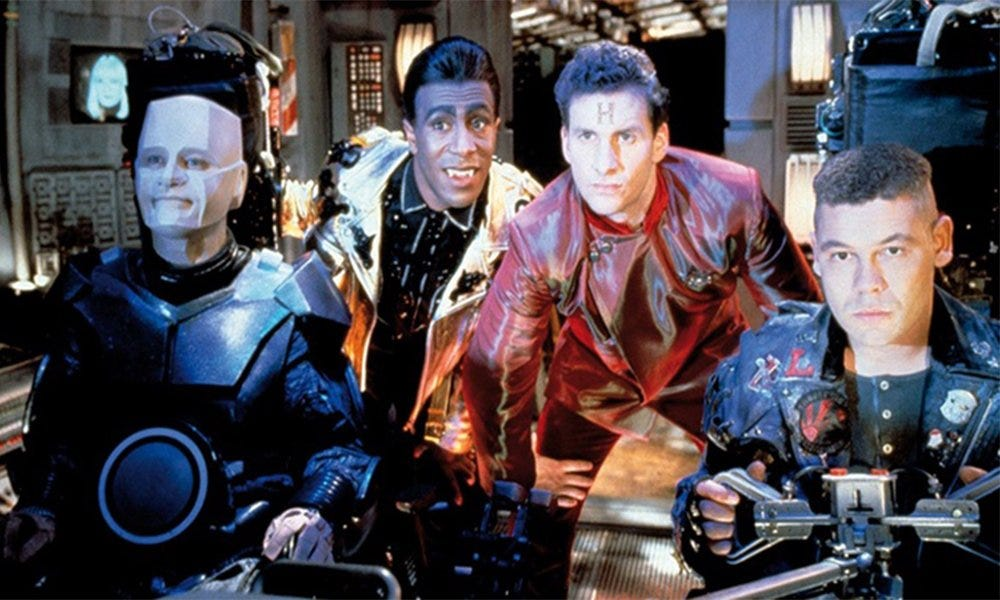So, you don’t want the AI to learn or converge too quickly. Instead of just predicting its best action, maximizing its reward, every time (that’s a greedy algorithm in comp.sci terms), you want the AI to initially explore the action space. Thus, with some decreasing probability epsilon, the AI will choose a random action from the action space, instead of its best prediction.

That’s all that the act method does, and that’s epsilon greedy learning. The rest of the time it picks the network’s best predicted action, simply by calling forward (i.e. running the current state through the network to predict the best next action).

Here’s the plain PyTorch network that does the job:


# env is the AI gym defined and instantiated above
class DQN(nn.Module):
def __init__(self):
super(DQN, self).__init__()
self.layers = nn.Sequential(
nn.Linear(env.observation_space.n, 20),
nn.ReLU(),
nn.Linear(20, 20),
nn.ReLU(),
nn.Linear(20, env.action_space.n)
)

def forward(self, x):
return self.layers(x)

def act(self, state, epsilon):
if random.random() > epsilon:
state = Variable(torch.FloatTensor(state).unsqueeze(0))
q_value = self.forward(state)
action  = q_value.max(1)
else:
action = random.randrange(env.action_space.n)
return action


As you can see, it has 3 inputs (each of which could be 0, 1, or 2, representing the current state) that fan out an input layer of 20 neurons with ReLU nonlinearity, a hidden layer of 20 neurons also with ReLU, reduced down to an output layer of 3 neurons which we will take the max of to predict the next action.

The hidden size of 20 is arbitrary. There’s minimal, but some, boilerplate:

• The state we have is just a single vector like [0,1,2]. PyTorch needs an tensor of N x num_features, where N is the batch size. Unsqueeze, effectively, turns [0,1,2] into [[0,1,2]] — that is, a batch size of one; one action predicted, given the current state, at a time. Conceptually simple but probably something you’d want to optimize for production, otherwise GPU capacity is being wasted.
• q_value, the output of a forward pass through the network, yields our expected rewards given each of the 3 actions we could take given a state. We find the max along the neurons’ axis (not 0, the batch axis), and that max() function gives a tuple, where  is the value and  is the index. Thus, if the forward pass yields Y=[-1.34, 0.62, 0.32] — Y.max(1) would be [0.62, 1] and Y.max(1) means the net suggests we take action 1. Y.max(1) suggests that the Q value if we took that action would be 0.62.

## Q-Learning

And so to the heart of the matter. Q-Learning is really just asking the question, “how to calculate loss at a given time step?” Working backwards from the end-game reward to see how our actions at each step contributed to the ultimate reward.

If you think back a few years to when Google bought DeepMind for a lot of money, the headlines represented that it was because DeepMind had some revolutionary way of solving Atari games, which led to AlphaGo and more recently, the phone-calling Duplex. Given what I know now, I don’t think that was the reason. Probably, they had a decent infrastructure via which they applied old-ish algorithms to a fancy problem domain, but more importantly, a great team that big G wanted to poach.

I have emphasized this before in my writing, but AI really advances just by people trying stuff, and it doesn’t have to be big companies, just someone with a GTX 1080 asking why it’s always been done via X when Y works better in practice.

So we come to the function _calc_loss_overepisode() mysteriously referred to above. The code just looks like this:


# gamma is a learning-rate hyperparameter, try 0.9
def calc_loss_over_episode(batch_size):
state, action, reward, next_state, done = replay_buffer.sample(batch_size)
state = Variable(torch.FloatTensor(state))
next_state = Variable(torch.FloatTensor(next_state))
action = Variable(torch.LongTensor(action))
reward = Variable(torch.FloatTensor(reward))
done = Variable(torch.FloatTensor(done))
q_values = model(state)
next_q_values = model(next_state)
q_value = q_values.gather(1, action.unsqueeze(1)).squeeze(1)
next_q_value     = next_q_values.max(1)
if done:
expected_q_value = reward
else:
expected_q_value = reward + gamma * next_q_value
loss = (q_value - Variable(expected_q_value)).pow(2).mean()

loss.backward()
optimizer.step()
return loss


The high level of this is:

• For each time step in a random sample of the batch (training is improved by not always moving through batches in the same order).
• Predict the Q values given the current state (expected rewards if the network took each of the 3 available Monty Hall actions, given the current state). Key to understanding here is that the network’s estimated Q values will start out completely random, but ultimately begin to converge to the real answer.
• Predict the Q values for the actual next state (again, they start off completely random) after we took the action predicted by the previous step.
• Discount the Q value a bit (multiply by gamma) before adding it to the expected reward. This is because in a stochastic environment, we can’t be 100% confident of the outcome of an action; gamma thus discounts future rewards a little compared to immediate rewards.
• Find the mean squared error (subtract ideal/expected reward from actual, square the difference and average it), then backprop and optimize the network parameters to minimize that loss (using SGD, Adam etc).

### Working it through

For the Monty Hall problem, imagine a batch was processed in order. Q(state, action) yields our next suggested action. It’s a vector consisting of the output neurons’ activations. Q([all doors closed], 2) is the activation of output neuron 2 after a single forward pass through the network with all inputs 0. It starts off as random garbage from an untrained neural network.

The network gets a 0.3 reward from the Gym environment for this step, and Monty opens a door. The neural net takes another step. Q([AI chose door 2 & Monty opened door 1], action) is calculated by another forward pass through the net with inputs 0,2,1. Our net is still garbage; let’s say the argmax of the output neurons this time is 1 (“open door #1”) with a value of 0.64. The stupid network tried to open the door Monty had already opened!

The gym environment now returns a reward of 0 and signals that the game has terminated (call it time T). Our loss function now says “my expected Q value was 0.64 [well, it is a vector so the other two actions would be included too], and I got reward 0. We can backprop that loss, which will help us to make a better guess as to the Q values (given a state) at time T-1. Which will then help to make a better guess about the Q values and actions to take at time T-2. (Except that in the learning step, time is flowing forwards so calculating the loss at step T-2 would predict an action/state/reward at T-1 and then time T).

One learning iteration just steps over two time periods, which we have randomly sampled from a batch. At some point, we’ll sample a terminal case (time T), which will give us a better idea of how the net’s action at the step before (T-1) contributed to the end reward. And then at some point we’ll sample that penultimate (T-1) move, which we now have a better idea about how it contributed to the end reward, and we can use that to get a better idea of the move before that (T-2), and so on, backwards to the start.

If that seems like cheating to you, just a little bit, then I agree: it’s just using big data to compensate for a complete lack of understanding and intuition. However, it does get you a nice graph that goes towards zero, as is the unstated goal of machine learning researchers:Loss per batch, ® Mean loss per frame (many batches)“)

For each batch (in the charts above I was using 32) of moves (which would likely include 16 completed games), you can see that the loss tends towards zero but doesn’t achieve it — because there’s still at least a 13 chance that the Monty Hall participant will lose. Sometimes the AI has a really unlucky round of games.

Meanwhile, back in the real world, the AI has indeed learned to switch its guess after Monty opens a door: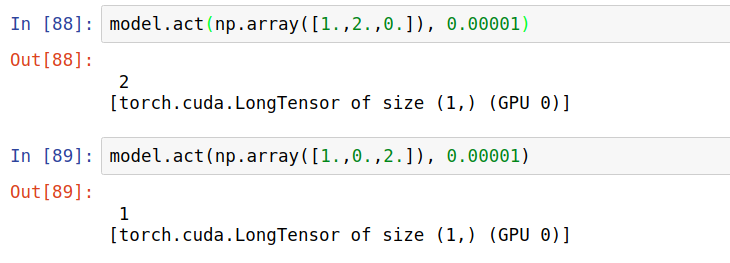My complete Jupyter Notebook for this is here.

## Next Steps

As with so much in AI, Deep-Q Learning has already been somewhat obsoleted, but if you can stand to be a little behind the curve this is a great repository of reinforcement learning stuff.

Just don’t forget that all this only works because we have the liberty of training our AIs over thousands of games; this network ‘solved’ Monty Hall with just 20 neurons, which is an order of magnitude fewer than the completely brainless roundworm.

That isn’t how the human brain works, so even a stout Bayesian should forgive Captain Holt for not ‘solving’ Monty’s game sooner. How should an AI learn when there are vastly fewer training examples; how should it meta-reason about a problem it encounters for the first time, as a first-time contestant on the Monty Hall gameshow would do? (Aside from the fact that gameshow contestants do not often seem to have a spare 20 neurons).

Nobody knows the answer to these questions — let’s keep going and figure it out!